# Investigating Periodic Variable Stars with a Robotic Telescope

Brody Beuker, Ethan Bowes, Tyson Brown, Rielly Castle, Kelly Christopher, Josh Fenwick, Joshua Foschia, Gurpiar Gill, Mayu Imamura, Zac Jalbert, Ivan Kocsis, Jessi Lipoth, Alex Magnus, Keaton Mollberg, Chidi Nwangwu, Alana Regier, Dawson Reid, Mikayla Rychel, Andrew Sotkowy, Amanda Stehwien, John Thuringer, Maia Wallis, and Daryl Janzen

Variable stars are extremely valuable in astronomy because when a star’s brightness varies there is some underlying physical cause making it happen—and when astronomers are able to explain the physical mechanisms driving such changes, we learn. In the course of the past decade alone, more than a million periodic variable stars have been discovered, mainly by survey missions designed to detect transient objects like near-Earth asteroids, supernovae, and exoplanets.

These periodic variables—such as the pulsating Cepheid and RR Lyrae variables that grow and contract at regular, periodic intervals, or eclipsing binary star systems that vary in brightness when each star eclipses the other from our perspective as they orbit a common centre of mass—hold valuable information that often cannot be determined in any other way. Mass, for instance, is determined through gravitational effects; therefore, it is really difficult to determine the mass of an isolated star, whereas the masses of stars in binary systems can be reliably estimated. And pulsating variable stars are important distance indicators that can be used e.g. to determine the distances to galaxies beyond the Milky Way, since their pulsation periods are related to their intrinsic brightness, or luminosity.

The reason why large surveys are so well-suited to detecting periodic variable stars is that these stars exhibit regular brightness variations. Therefore, they do not need to be observed continuously since sporadic observations over many periodic oscillations in brightness can be folded into a single phased light curve (see Figure 1). These surveys, however, often observe light through one or two wavelength bands, where more are required to be able to tell a star’s whole story (e.g., measure its temperature variation throughout the period), and in the case of infrared surveys these observations are made in wavelength bands where photometric techniques are not as well-refined as the techniques of optical astronomy. Furthermore, the classifications of these large numbers of targets are mainly made through modelling and statistical techniques, based on a limited number of observations, and require more data and analysis to determine their variability type as well as physical characteristics.(a)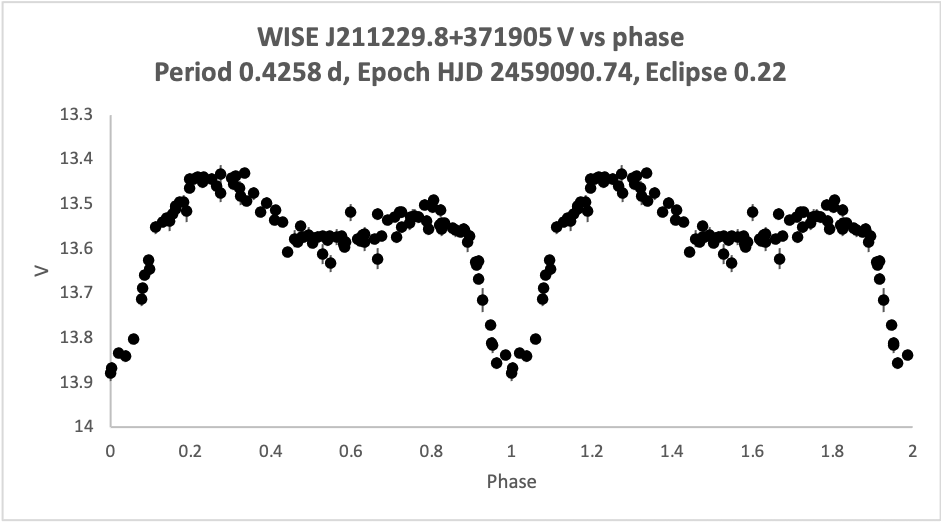(b) Figure 1: V magnitude (brightness) data for star WISE J211229.8+371905 plotted (a) with respect to heliocentric julian date (essentially, a running date used in astronomy, corrected for effects of Earth’s movement around the Sun, and (b) the same data, folded onto a period of 0.4258 days and plotted over two periods of brightness fluctuation. The light curve in (b) indicates that this star is an eclipsing binary system, with the brighter component eclipsed at phase 0 and 1, with the dimmer component eclipsed at phase 0.58, and with rotational variability possibly due to star spots causing continuous variation throughout the period.

In this post we present the results of a photometric study carried out by the University of Saskatchewan Astronomy 213 class during the fall of 2020. All data were collected with the Skynet PROMPT-USASK robotic telescope, which consists of a 0.41-m f/11.2 Ritchey-Chretien OTA and an FLI ML4710 Broadband CCD camera, which is fully automated through the Skynet Robotic Telescope Network and operated on a Planewave L-500 direct drive mount. Data were collected with BVg’r’i’ and z’ photometric filters between August and November 2020, with raw image calibration handled automatically by Skynet. Following data collection, images were processed through the Our Solar Siblings Pipeline, to measure raw brightness counts for all stars in a target field using 3 point spread function-based and 3 aperture-based methods, and data were subsequently processed using astrosource, in order to calibrate the magnitudes (brightnesses) of all stars in the field by referencing standard catalogues, detect variable stars, and statistically estimate the most likely periods of periodic variables.

The Wide-field Infrared Survey Explorer (WISE) Catalog of Periodic Variable Stars (Chen et al. 2018) served as a basis for our project, as we targeted stars that were first discovered in that study, which were classified as RR Lyrae (short period, pulsating) variable stars. In total, we monitored 16 short-period variable stars, of which 4 were eventually classified as RR Lyrae and 12 were classified as eclipsing binary or other systems.

#### WISE J201217.1+462903 and GSC 03559-00741 by Brody Beuker and Ethan Bowes

The two stars we observed were WISEJ201241.6+463313 (Variable 1) and GSC 03559-00741 (Variable 2). Variable one is located at Ra: 303.0713821 Dec: 46.4842706, while Variable 2 can be found at Ra: 303.1733181 Dec: 46.5537767 (see Figure 2). Chen et al (2018) classified both variable 1 and 2 as an RR Lyrae variable star. The data collected in the present study showed that while both stars are clearly periodic variables, only one of these classifications is actually correct.

Variable 1

We collected parallax data from the GAIA catalog and used this to determine the distance of the target star. We determined this distance to be 994.26 +/- 26.77 parsecs. From the B and V magnitude versus period relationship plots (see figure 3),  and using theory described in Drake et. al we can determine that Variable 1 is a contact binary star system by looking at the shape of the curves. We can see a clear cycloidal pattern in these curves. It appears to have an eclipse of 0.28 days and a period of 0.6351 days. We also generated graphs of the temperature. The temperature (see figure 4) on the other hand does not appear to resemble the magnitude graphs, as it appears more linear and varies from approximately 5200 to 5900 degrees kelvin.

Variable 2

The parallax data we collected was used to determine the distance of the target star. We determined this distance to be 1489.48 +/- 63.56 parsecs. From the B and V magnitude versus period relationship plots,  and using theory described in Drake et. al (2014) we can determine that Variable 2 is an RR Lyrae of the type ab, by looking at the shape of the curves (see figure 5). We can see a clear pattern in these curves with a sharp rise, followed by a slower descent over the period creating the well known “saw tooth” look. In this case the rise is 0.27 days or 32.1% of the period of this star. The temperature of this star (see figure 6) appears to vary with the period in the same shape and at the same point as the magnitude along its period. The last thing we calculated for this star was the radius and it appears to be approximately 15 times the size of the sun.

#### WISE J040050.2+432116 By Tyson Brown and Josh Fenwick

WISE J040050.2+432116 was classified as an RRab Lyrae with a period of 0.6849766 days by Chen et al (2018). Through collecting and analyzing data, we found the classification on the AAVSO VSX to be incorrect. A phased light curve, if correct, (shown below in Figure 8a) shows that WISE J040050.2+432116 could actually be a partially eclipsing contact binary system with a period of 1.36938 days, twice that found in Chen et al (2018). Using tests outlined in Drake et al (2014), designed precisely for situations such as these, namely the M and V-w1 tests, we were able to conclusively say our classification is correct.(a)(b)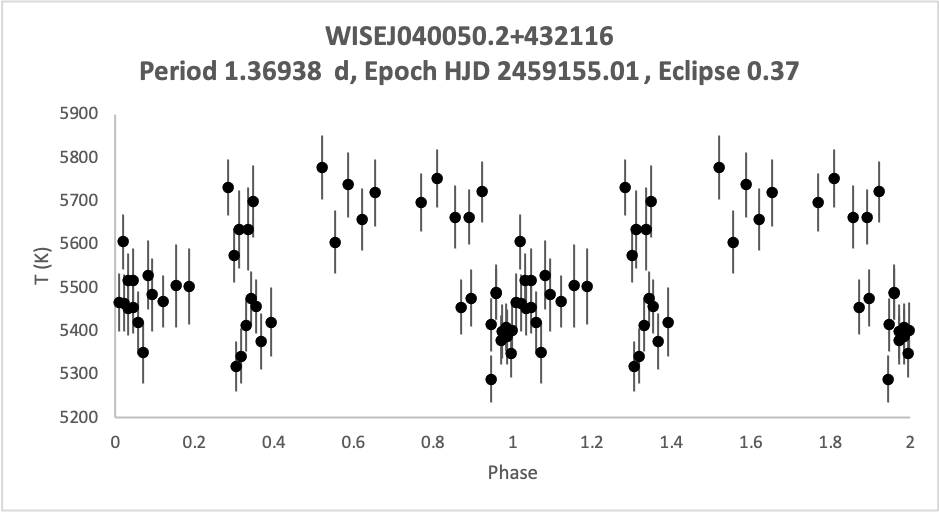(c)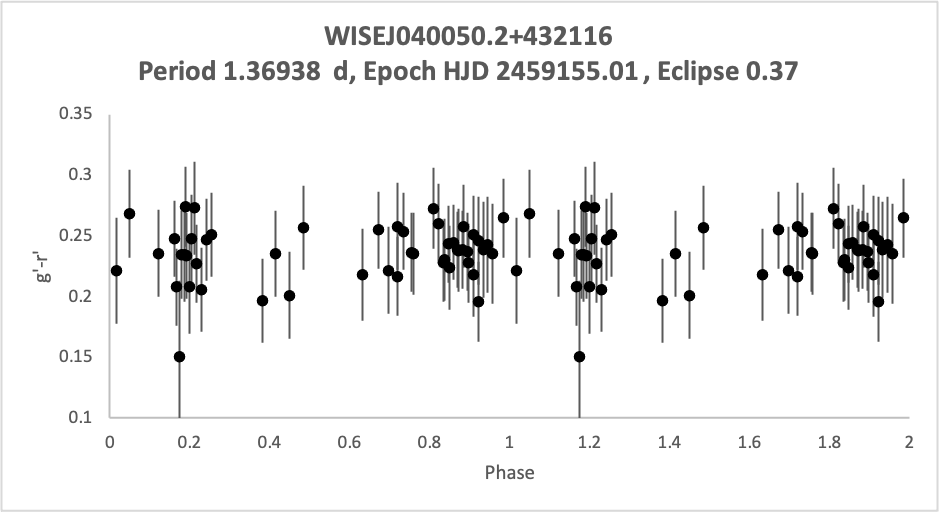(d)
 Figure 8: plots of gathered WISE J040050.2+432116 data. (a) an r’ magnitude phased light curve, (b) plot of luminosity vs phase (c) A plot of the temperature found using Ballesteros’ formula (d) the g’-r’ magnitude plotted versus phase.

Using our photometric data along with Gaia DR2 parallax data we found a distance of 1209.9±68.8 pc. The luminosity of the system (Shown above in figure 8b) fluctuates between 120 and 160 times the luminosity of the sun with an average of 140. This shows our binary system to be made of two quite massive giant stars. Plotting of the temperature (Shown above in figure 8c) found using the formula found in Ballesteros (2012) resulted in scatter that we couldn’t account for. Though if we plot g’-r’ (Shown above in figure 8d) we found that the magnitude and temperature to both remain relatively consistent.

#### WISE J230703.9+414254 by Rielly Castle and Dawson Reid

WISE J230703.9+414254 is a variable star spotted by the WISE survey in 2018. This star is located at right ascension: 346.76652 and declination +41.71519 in the constellation of Andromeda. The WISE survey had initially categorized as an RRc Lyrae variable with a period of 0.3469 days. In our research, we found that the predictions made about this star were incorrect. Our initial analysis has shown that  WISE J230703.9+414254 is, in fact, a contact binary system with a period of 0.6936 +/- 0.0018 days. The varying maxima of each star’s peak (Figure 9) implies that this system is undergoing the O’Connell effect as well (Drake et al, 2014).Figure 9

Digging deeper into this star system, we found that the B-V index had a value of 0.437 +/- 0.44, which corresponds to an average effective temperature of 6799 +/- 117 K using Ballesteros’ (2012) equation. Applying this information, we were able to determine that the average luminosity of WISE J230703.9+414254 is around 27 times that of the sun (Figure 10).Figure 10

After finding out that WISE J230703.9+414254 is a binary star system, we were able to conduct some brief analysis to determine different characteristics of the star system. The first feature we found was the semi-major axis using Kepler’s 3rd law. With this, we found that the semi-major axis is 3.299 +/- 0.062 solar radii. This value can be further defined to be the radius of the orbit, as we can approximate that the eccentricity is 0 due to the system being a contact binary. Lastly, we were able to find that the net mass of the WISE J230703.9+414254 star system is  1.000 +/- 0.063 solar masses. Any additional information such as radial velocities or inclination will need to be acquired by the use of computational modeling and spectroscopy.

#### WISE J183729.6+291355 and WISE J183753.4+291113 by Kelly Christopher and Alana Regier

The variable star WISEJ183729.6+291355, located in Lyra, was assumed to be an RRab by Chen et al (2018).  The star    The approximated period was 0.6965317 days.  By analyzing the light curve of this star, it does not appear to be an RRab.  Another variable star (WISE J183753.4+291113) also appeared in the field, but it was too dim in comparison to the primary target star to accurately analyze it.

The phased light curves processes from the second sets of observations appear to have a more specific shape than the light curve from the preliminary observations. The light curve from the second set of analysis appears to have double the period that was initially suspected of WISEJ183729.6+2913, which can be seen in Figure 12. The period was initially thought to be 0.6965317 days, but due to the varying magnitude of the peaks, the suspected period was changed to 1.387577 days. The light curve retains the sinusoidal shape that appeared in the initial results.

The distance from Earth to the primary target star was determined using the GAIA DR2data from Aladin. WISEJ183729.6+291355 was determined to be 1905.4 parsecs away, with a distance error of 171.7 parsecs.

Using the (B−V) data, the temperature of the star was calculated using the Ballestros formula and then was plotted as a function of phase. The temperature ranged from 6838K to 7222K,but had no observable trend and appeared randomly distributed, as seen in Figure 13.

An interesting feature of WISEJ183729.6+291355 is that it appears to be exhibiting the O’Connell effect. The O’Connell effect occurs when the two maxima and minima in the lightcurve of a contact binary are at uneven magnitudes. This is likely caused by ”star spots, gas stream impacts, and circumstellar matter”, according to Drake et al (2014). The O’Connell effect is a feature of contact binaries, which is another indication that the star is not an RRc.

Due to the lack of observations, the identity of WISEJ183729.6+291355 cannot be determined with any certainty, as there are simply too many gaps in the data to confirm some of the observed trends. Our research suggests that the star may be an EW or EB type eclipsing binary or contact binary exhibiting the O’Connell effect, however it cannot be definitively ruled out as an RRc Lyrae type variable star. It’s extremely unique phased light curve warrants more research, as it shows similarities to other previously unclassified variable stars.

#### WISE J184427.5+302358 and ZTFJ184419.13+302638.5 by Joshua Foschia and Mayu Imamura

WISEJ184427.5+302358

The WISEJ184427.5+302358 is a star located at a right ascension of 18:44:27.597 and a declination of +30:23:58.45 and is part of the constellation lyra. This star was classified as a detached binary or also known as an EA star system. The WISEJ184427.5+302358 meets all the standards of a detached binary and is very visibly a detached binary from both of its B magnitude vs phase plot shown in Figure (14a). The period for this star was determined to be 1.0909 days and the average luminosity with respect to the sun is 10.667. In addition, the range in temperature can be seen between 6475 K and 7190 K (Figure(14b)).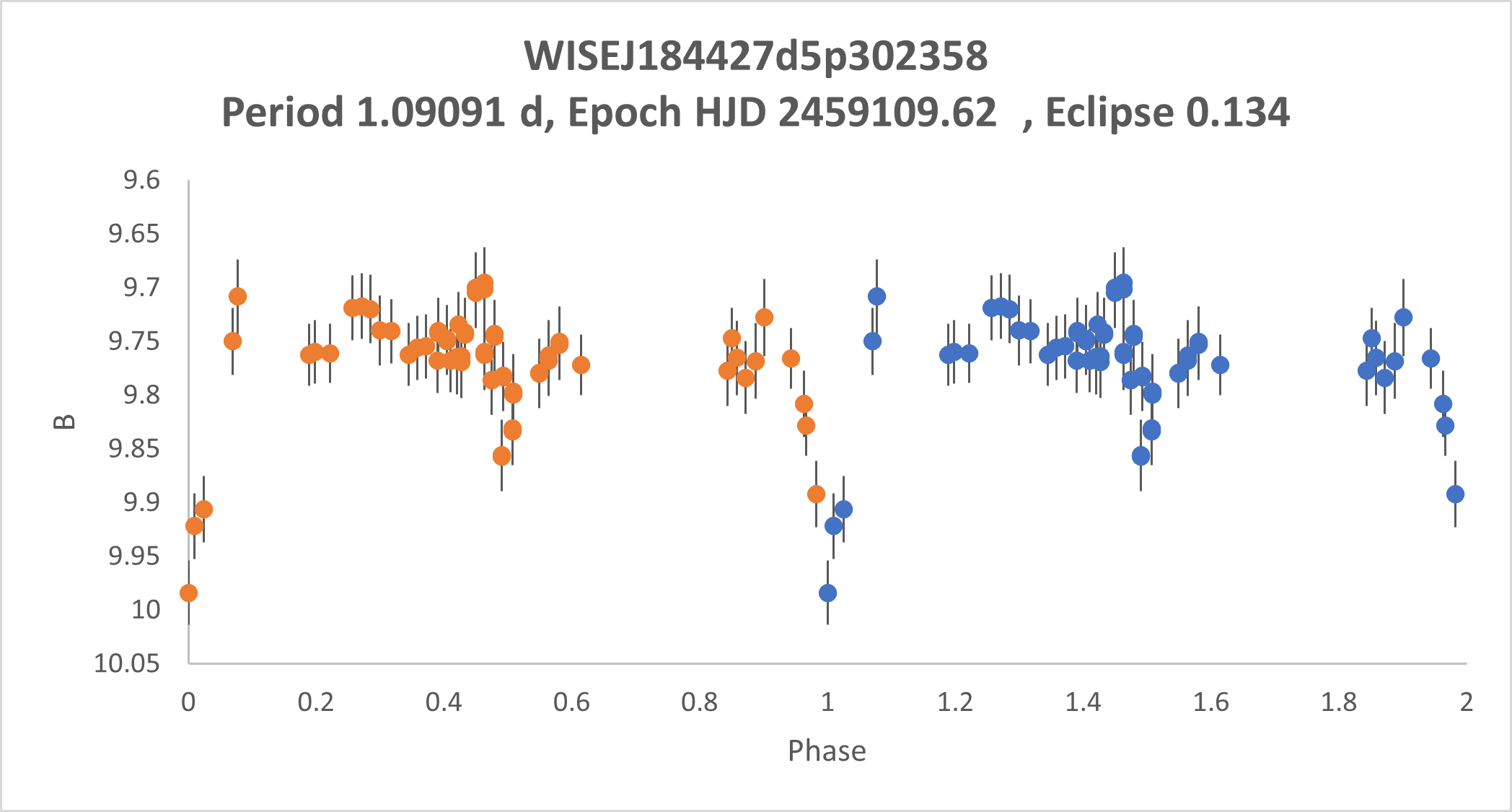(a)(b) Figure 14

ZTFJ184419.13+302638.5

ZTFJ184419.13+302638.5 was discovered by the Zwicky Transient Facility and is a star located at a right ascension of 18:44:19.13 and a declination of +30:26:38.5 in the Lyra constellation. This star was classified as a contact binary or also known as an EW star system. Contact binaries and RRc’s have very similar absolute magnitude vs. phase curves. In addition to the curve seen in Figure (15a), the temperature also had to be taken into account to classify the star correctly. RRc’s normally have a temperature range of around 6500-8000k, however, the ZTFJ184419.13+302638.5 star ranges around 5000-7250K (Figure (15b))  and was therefore not classified as a RRc. The period for this star was found to be 0.55440 days and the average luminosity with respect to the sun is 14.347.(a)(b) Figure 15

The classifications of both of these stars are in line with AAVSO’s listed classifications. An EA star system for the WISEJ184427.5+302358 and a EW star system for the ZTFJ184419.13+302638.5, however, both of these stars where re-classified during this study as before the study WISEJ184427.5+302358 was classified as a RR Lyrae on AAVSO and ZTFJ184419.13+302638.5 was classified as a RRc on AAVSO.

#### WISE J172508.1+371155 and WISE J055815.4+303147 by Ivan Kocsis and Amanda Stehwien

Two observations were done over the course of the semester. WISE J172508.1+371155 and WISE J055815.4+303147. WISE J172508.1+371155 is located at a right ascension of 261.284 and declination of 37.19883 as seen in figure (16b). WISE J055815.4+303147 has a right ascension and declination of 89.56441 and 30.5299 as seen in figure (16a). Each one of these observations included one star that was analyzed.(a) (b)

Figure 16 : Positions and names of the target stars in their respective star fields as viewed from the Prompt-USASK telescope.

The analysis of the stars was done by utilizing Astrosource to gather information from the collected observations. from the information collected, quantities could be determined and plotted. These quantities will aid in the determination of the type of star being observed.  Some of the plotted quantities include:

• Distance, calculated from parallax measurements available in GAIA catalog on Aladin
• for 838.5 +- 36.98 pc for WISE J055815.4+303147, and 1002 +-20.48 pc for WISE J172508.1+371155.
• Temperature using The Ballesteros’ formula
• Luminosity, found using the absolute magnitudes
• Radius of the star is calculated using the Stefan-Boltzmann law
• Catelan analysis (on suspected RR lyrae)

plotting the magnitude of the brightness in the B filter provides us with a light curve that can help determine what type of star the object is. In Figure 17b, the curve demonstrates a sinusoidal shape. In Figure 17a, the function of the shape is more complex.(a)(b)

Figure 17

Comparing the shapes of the resulting graphs from the data collected (such as Figure 17), to the data presented in the literature, we can conclude that the star in observation WISE J172508.1+371155 in most-likely a RR lyrae of type RRc. The slight drop, followed by a slight rise, followed by another more dramatic drop seen in Figure 17a is characteristic of a contact binary star. In particular, it is most likely a binary system where one of the stars mass is much larger than the other, resulting in the asymmetric look in the graph.

#### WISE J185000.4+643033 and WISE J213339.0+295713 by Alex Magnus and Jessi Lipoth

WISEJ185000.47+643033.7 is located in the Draco constellation at RA 282.50196 and Dec: 64.50937. According to the data from the WISE catalog, the star has B magnitude of 10.3  a V magnitude of 9.90, and a period previously measured at 0.7621 days (Chen, 2018). It is not currently designated as a variable star on SIMBAD, but is listed as an RRab variable on AAVSO. The data we collected in this study seems to indicate that the object is not, in fact, an RRab.

Figure 18a shows the light curve we generated in B for WISEJ185000.47+643033.7. This light curve immediately suggests that the object is an eclipsing binary star system, as opposed to an RRab. The system clearly experiences eclipses that occur in two forms: a deep, V shaped primary eclipse and a smooth, shallow secondary eclipse that occurs in between primary eclipses. These continuously alternating eclipses of uneven depth suggests that the object is a semi-detached binary system. The rise left of the minimum also reaches a greater height than the opposing side, which indicates that the star has a fairly elliptical orbit.

Figure 18b shows a temperature graph for WISEJ185000.47+643033.7. As one can see, the temperature remains fairly consistent – if a little scattered – over the course of its period. This, coupled with the fact that the secondary eclipses are so shallow, suggests that one of the stars in the binary system is significantly smaller than the other.

We determined the star’s B magnitude to be 10.3, the V magnitude to be 10.0,  the average luminosity to be 12.5 times that of our Sun, and the period of the star to be 1.519 days – which is approximately double the period listed in the WISE catalog. This value makes sense:  often, eclipsing binaries can be mistakenly detected as RR Lyraes at their half-periods, since half of a binary light curve period can often look similar to a full-period RR Lyrae light curve.(a)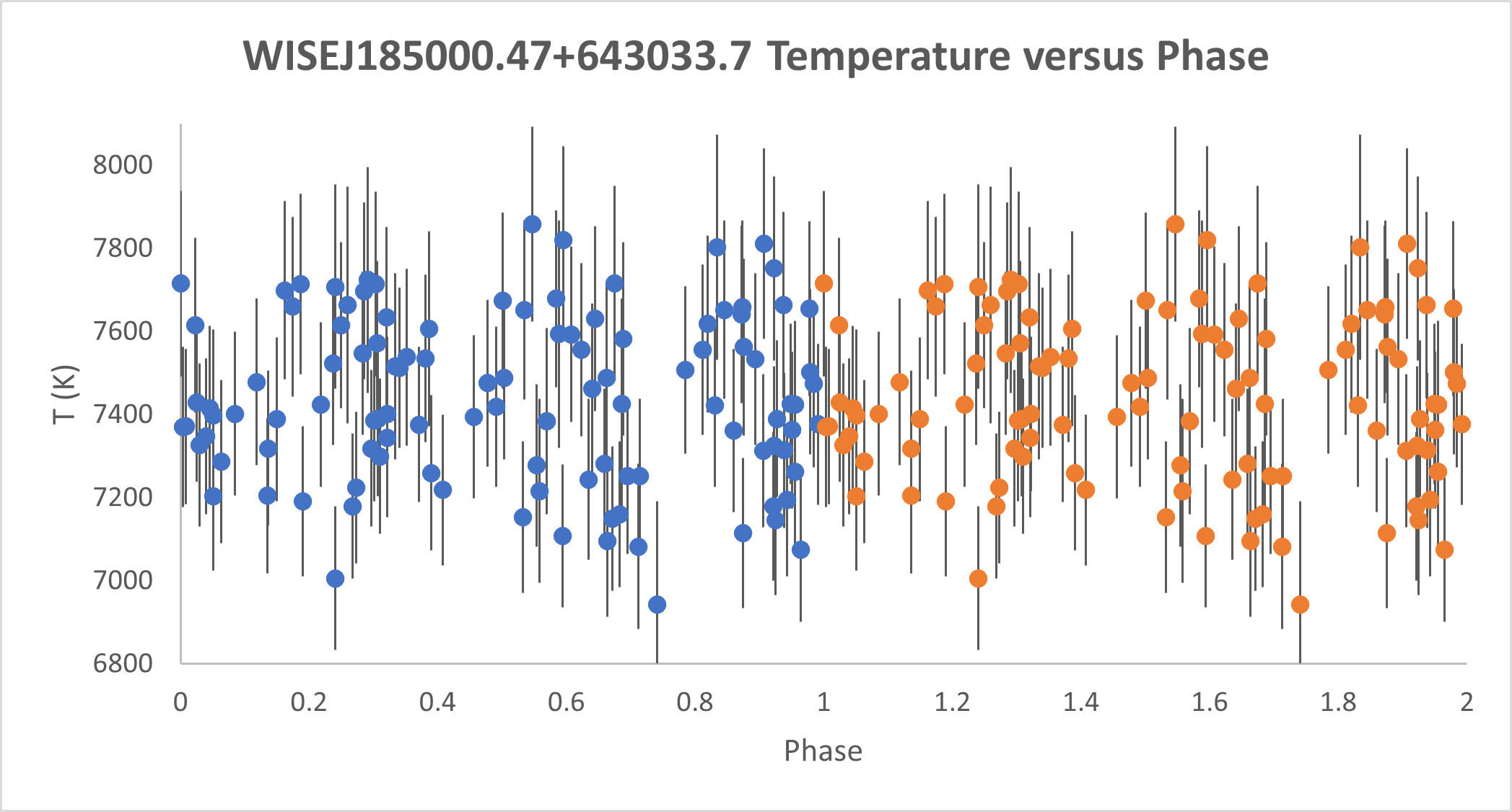(b) Figure 18: a) B magnitude (brightness) data for star WISEJ185000.47+643033.7 plotted with respect to the phase and (b) The temperature of WISEJ185000.47+643033.7 with respect to the phase.

WISEJ213339.0+29571 is located in the Cyngus constellation at RA: 323.41262 and Dec: 29.9537. The WISE catalogue lists the object’s period as 0.3817 days. Though it was classified as an RR Lyrae by the WISE catalogue, it is designated as an eclipsing binary system on both SIMBAD and AAVSO. Our data seems to indicate that WISEJ213339.0+29571 is, in fact, an eclipsing binary.

Figure 19a shows the light curve in B for WISEJ213339.0+29571. The sinusoidal shape of the light curve suggests two possibilities: an RRc variable or contact binary system. We believe it is a contact binary for two reasons:

• We determined the absolute V magnitude of this object to be roughly 2.0; we also determined that its temperature ranges between 5800-6400 K. RRc variables are expected to be located within the instability strip of an H-R diagram, which corresponds to an absolute V magnitude between 0.3-1.2 and temperature between 6500-8000 K. Therefore, the V magnitude for this star is too large to be an RRc, and its temperature is too low.
• We applied a color test used for determining whether a star is an RR Lyrae or a contact binary. For this test, we subtracted the V magnitude and the w1 – or infared – magnitude. This yielded a value of 1.37. According to Drake et. al, this would put the star above the expected V-w1 range for an RR Lyrae (Drake et al, 2014), meaning it is most likely a contact binary.

Figure 19b shows the temperature vs. phase plot for WISEJ213339.0+29571. As can be seen in the plot, WISE WISEJ21’s temperatures increases slightly as the secondary eclipse occurs, despite both eclipses being similar in size. Since this is a contact binary system, this effect likely comes from one of the stars being somewhat hotter than the other. Thus, the temperature peaks when it is face on with the observations. This effect is very subtle due to the close intermingling of stars found in contact binaries.

We determined the period of this star to be 0.762 days. Once again, this is approximately double the period listed in the WISE catalogue. Like WISEJ185000.47+643033.7, this star was also likely mistakenly detected as an RR Lyrae at its half-period. We also determined the star’s B magnitude to be 11.3, the V magnitude to be 10.72,  the average luminosity to be 10.5 times that of our Sun.(a)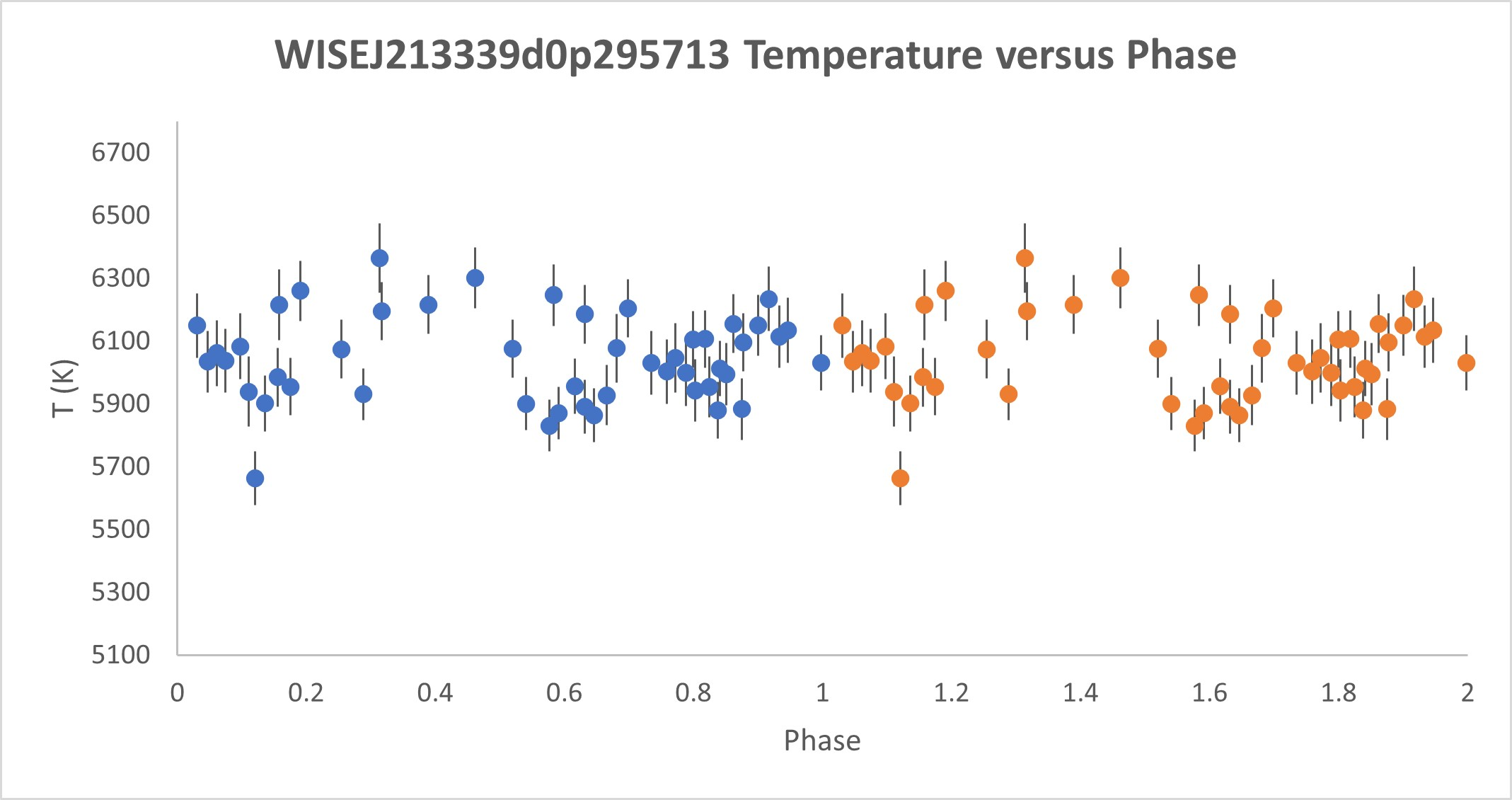(b) Figure 19: a) B magnitude (brightness) data for star WISEJ213339.0+29571 plotted with respect to the phase and (b) The temperature of WISEJ213339.0+29571 with respect to the phase.

#### WISE J202551.1+451702 and WISE J215202.8+223429 by Keaton Mollberg and Andrew Sotkowy

WISE J215202.8+223429, known as AV Peg, is found at the J2000 coordinates of RA 328.012 and Dec +22.574, in the constellation of Pegasus. Chen et al (2018) classified AV Peg as an RR Lyrae in the WISE catalog, with a period of 0.391 days. Additionally, it was first discovered and classified as an RR Lyrae in 1931 (Udovichenko, 2007), and it has since been a subject for over 153 papers. The absence of recent CCD photometric studies of AV Peg motivated our choice of this target.

Figure 21(a) shows the phased lightcurve of AV Peg in the SDSS filter of r’, which had the most data points due to preliminary observations. Astrosource confidently predicted that the period of AV Peg is 0.39002 days (with a small error range). The phased lightcurve in Figure 21(a) shows the distinct shape of an RRab variable, with a sizeable magnitude difference of ~0.9 in r’. The temperature versus phase plot (Figure 21(b)) was formed by using the black body temperature equation formulated by Ballesteros (2012), and it shows that the temperature of AV Peg fluctuates with the same pattern as the lightcurve. The lightcurve and temperature plots confidently suggest that AV Peg is an RRab with a period of 0.39002 days, which agrees with WISE’s variable classification and period prediction (within error).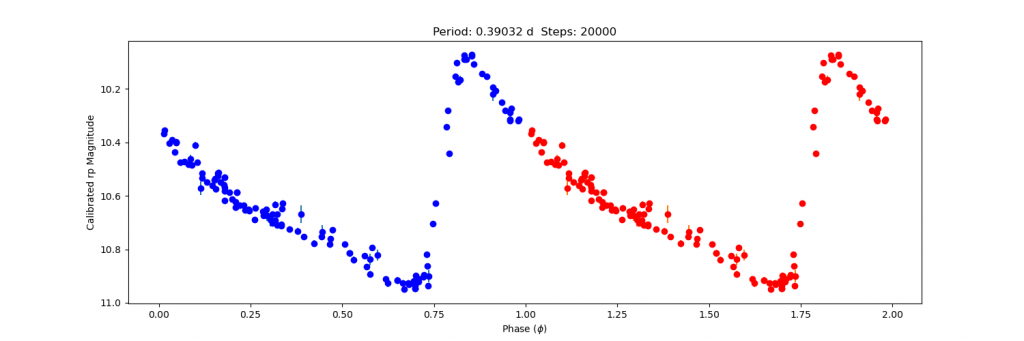Figure 21(a): Phased lightcurve in r’ of WISE J215202.8+223429Figure 21(b): Temperature vs. phase of WISEJ215202.8+223429, using B and V magnitude data in Ballesteros’ Equation (2012)

WISEJ202551.1+451702 is found in the constellation of Cygnus at 306.463 +45.284 (J2000). WISE classified this star as an eclipsing binary (EB), with a half-period of 0.554 days. This star has not been studied before, according to SIMBAD and AAVSO VSX who have classified the star as a non-variable and an EB, respectively.

Upon initial tests of data in all six filters, astrosource’s period prediction was evenly split between two periods: the first period was 1.108 days (twice that of WISE’s predicted period), which created a lightcurve that is reminiscent of an EB’s major minimum, albeit with a large data gap where the second minimum would occur (Figure 23(a)). The second period of 1.245 days formed a lightcurve that indicates a long-period RRab or RRc, with a relatively steep increase and a small bump during the decline at ~0.45φ (Figure 23(b)). It’s also important to consider that WISE’s predicted period of 0.554 days (half of astrosource’s predicted period of 1.108 days) also created a sinusoidal lightcurve that is reminiscent of an RRc, an eclipsing binary system, or a rotational variable such as an RS CVn binary system (Figure 23(c)(i)).

In an attempt to add more evidence for these possible periods, we utilized data from the ASAS-SN catalog of variable stars (Jayasinghe, 2018) for this star, which consists of magnitude data in V. The various periods found by astrosource and WISE were imposed onto the data to form phased lightcurves to see if the lightcurve would resolve. The period of 1.245 days was the only period to successfully make a pattern out of the ASAS-SN V data, where the period was further refined to 1.24524 days to create a sinusoidal lightcurve (Figure 23(b)(ii)). This new refined period was also imposed onto our own collected data to compare the curves (Figure 23(b)(i)).(i)(ii) Figure 23(a): Phased lightcurve of WISE J202551.1+451702 at a period of 1.108 days with (i) our collected data in B, and (ii) ASAS-SN catalogued V data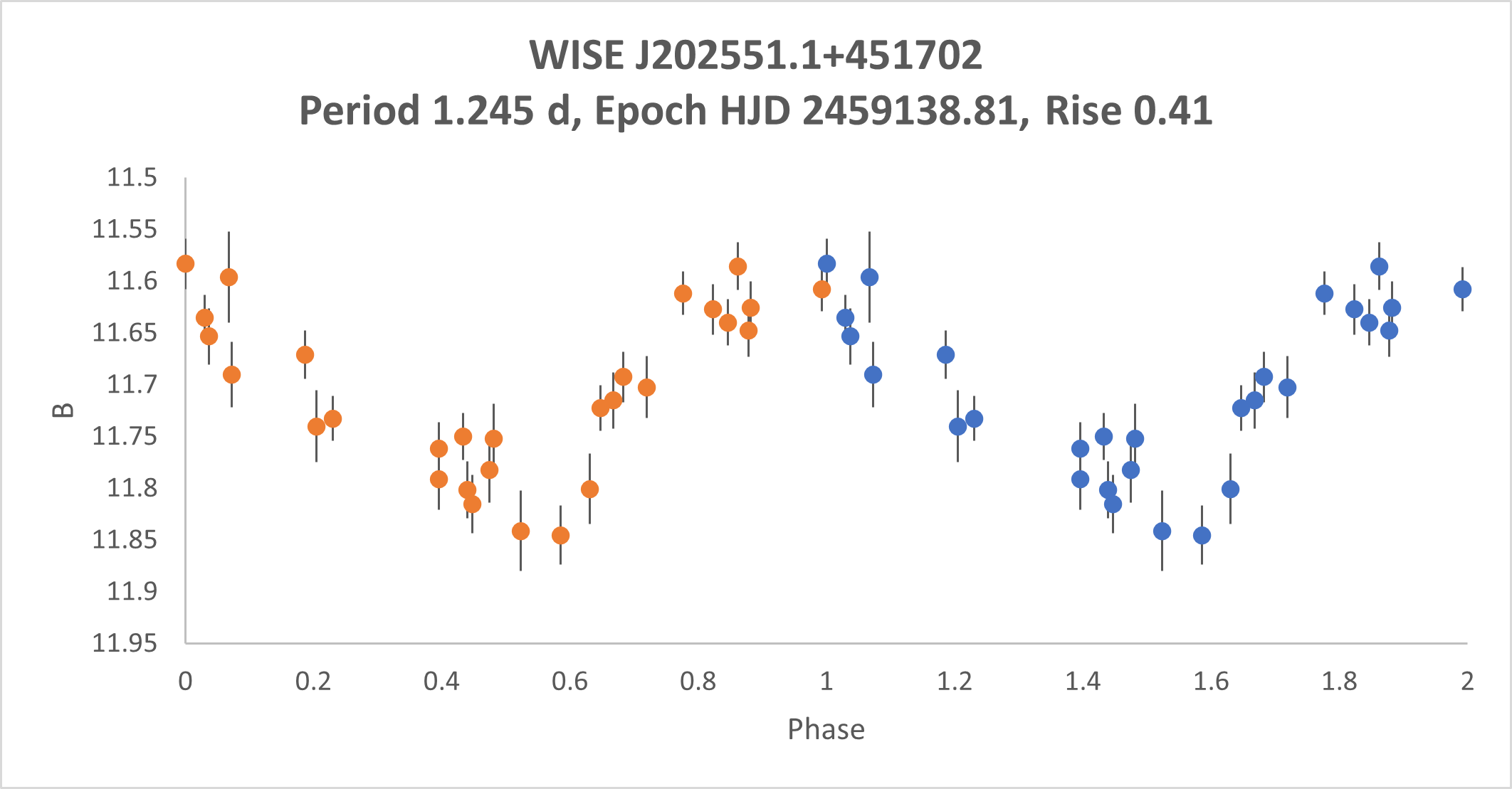(i)(ii) Figure 23(b): Phased lightcurve of WISE J202551.1+451702 at a period of 1.24524 days with (i) our collected data in B, and (ii) ASAS-SN catalogued V data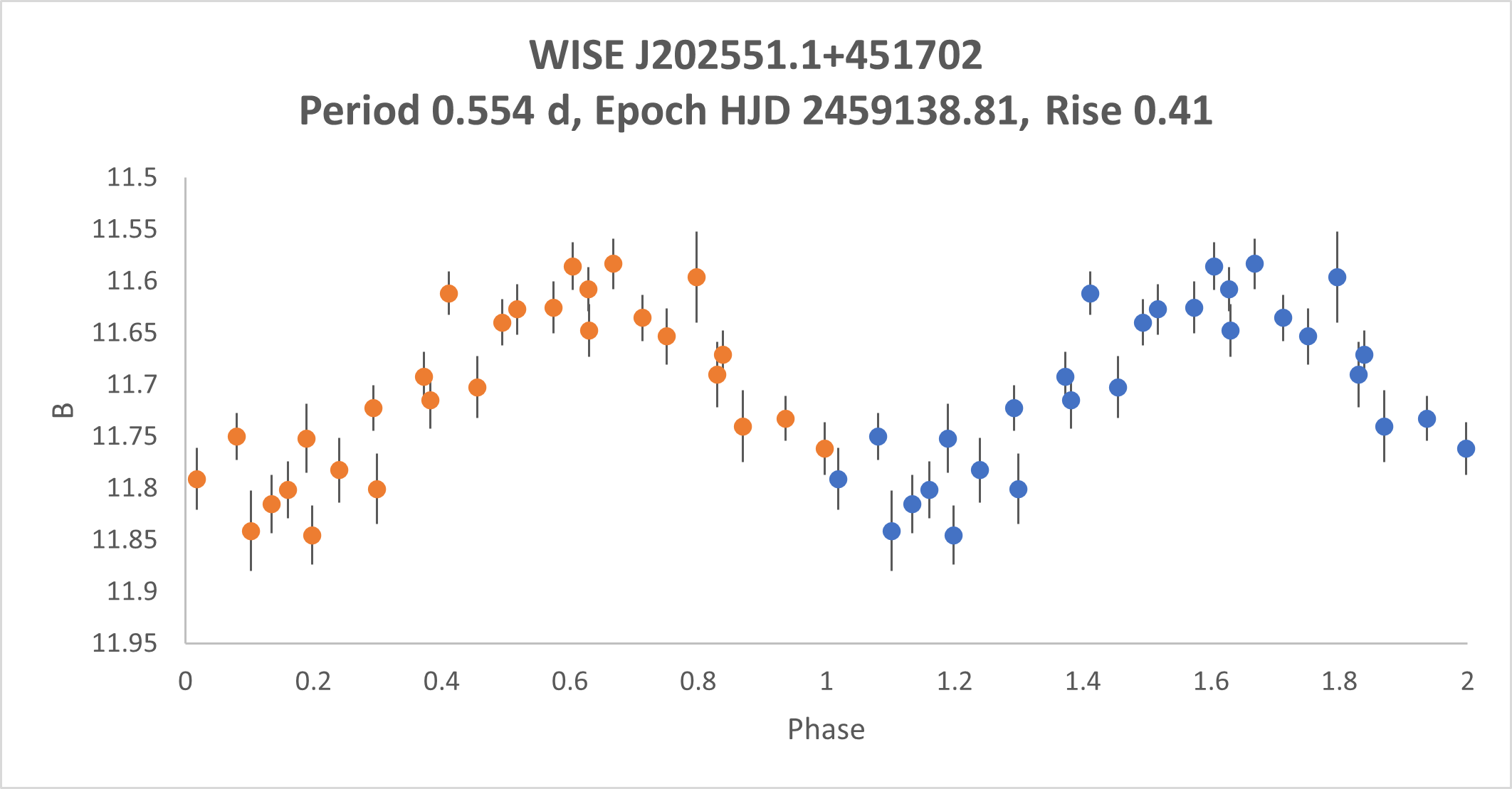(i)(ii) Figure 23(c): Phased lightcurve of WISE J202551.1+451702 at a period of 0.554 days with (i) our collected data in B, and (ii) ASAS-SN catalogued V dataFigure 24: Temperature vs phase for WISE J202551.1+451702 with a period of 1.24524 days. Graphs using alternate periods showed similar scatter with no obvious patterns.

The temperature vs phase graphs, regardless of period, did not show a clear trend (Figure 24). However, the graph revealed that WISE J202551.1+451702 has a temperature range of 5500K-6200K. This is typical of an F-type or G-type main-sequence star, such as an RRab or rotational variable, but too cool for a typical RRc (Drake et al, 2014). The period is also much longer than a typical RRc, who often have observed periods of <0.5 days (Drake et al, 2014). Also, the lightcurve amplitude of ~0.3 magnitudes in V is a plausible amplitude for an RRab, and typical of rotational variables (Drake et al, 2014).

With this new data now organized, the search for a classification of WISE J202551.1+451702 was much more narrowed. For the possible period of 1.108 days, predicted by astrosource (Figure 23(a)), the large gap in data is concerning, considering that observations were taken over two months. The scatter of the ASAS-SN plot also makes 1.108 days an unlikely period. The period of 1.24524 days (Figure 23(b)) is most promising, as it was a period predicted by astrosource and it forms periodic lightcurves for both sets of data. The case of 0.554 days, as predicted by WISE (Figure 23(c)), is also plausible as both lightcurves are reminiscent of a rotational variable, due to the linear rises and declines. However, the large amount of scatter in the ASAS-SN data, and the absence of this prediction by astrosource, makes the prediction dubious.

Thus, we concluded that WISE J202551.1+451702 is likely a long-period RRab or a rotational variable with a period of 1.24524 days. This conclusion is not a confident one, but it nevertheless calls for more research and a reclassification for AAVSO VSX and SIMBAD. Further photometric observations would further strengthen period predictions, improving the accuracy and trustworthiness of a lightcurve classification. Subsequent spectroscopic observations would also be useful to determine if WISE J202551.1+451702 is a rotational variable, and what specific variety it may be—scanning for chromospheric activity as indicated in section 6.7 of Drake et al (2014) would assist in making a confident classification of WISE J202551.1+451702.

#### WISE J192139.8+102257 and WISE J215550.8+571948 by Chidi Nwangwu and John Thuringer

Chen et al (2018) classified WISE J215550.8+571948 as an RRc Lyrae variable (see Figure 25). Photometric observations and analysis in this study have shown that it is likely a W Ursae Majoris (W UMa) contact binary system.

• The r’ magnitude phased light curve (Figure 26a ) exhibits a shape characteristic of a W UMa star with a varying eclipse depth due to its degree of contact and inclination and a continuous light variation.
• The temperature plot (Figure 26b ) demonstrates a low degree of fluctuation throughout its period that is consistent with contact binary systems where mass flows from one component star to another equalizing their temperatures over time.
• The g’-r’ colour plot (Figure 26c ), which provides comparable information to the B-V values used with Ballesteros’ formula due to the similarity in passbands, also presents the same low degree of fluctuation as found in the temperature plot.
• Four additional identification techniques outlined in Drake et al (2014) were also employed (M test statistic, amplitude – period distribution, V-w1 – period plot, and u-g vs g-i colour-colour plot), all yielding results compatible with an eclipsing binary system.(a)(b)(c)(d)
 Figure 26: (a) r’ magnitude phased light curve, (b) temperature plot, (c) g’-r’ colour plot and (d) PDM period likelihood plot for WISE J215550.8+571948

WISE J215550.8+571948 was found to have a period of 0.829 days, a light amplitude of 0.21 in the V band, and a luminosity approximately 200 times that of our Sun. Utilizing Gaia parallax values, the photometric data yielded an estimated distance of 988 light years. Temperatures indicated a late F or early A spectral class.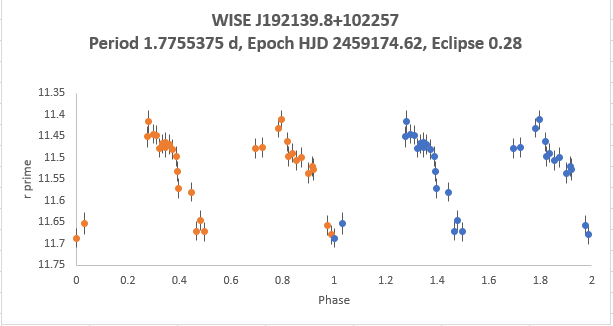(a) r’ magnitude phased light curve for  WISE J192139.8+102257(b) temperature plot for  WISE J192139.8+102257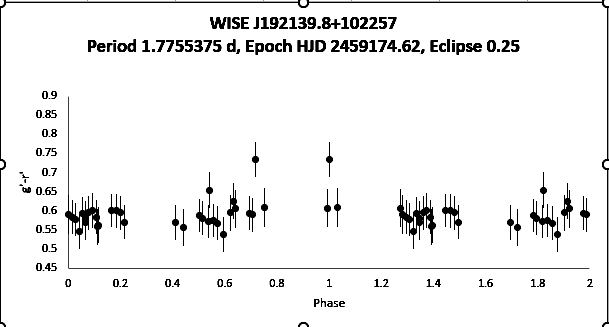(c) g’-r’ colour plot for  WISE J192139.8+102257(d) PDM period likelihood plot for  WISE J192139.8+102257

Figure 27: WISE J192139.8+102257 was found to have a period of 1.78 days, a light amplitude of 0.429 in the V band. Utilizing Gaia parallax values, the photometric data yielded an estimated distance of 3163 light years.

#### WISE J202625.0+334224 and ZTF J202615.90+334024.7 by Mikayla Rychel and Maia WallisFigure 28. A DSS2 colour image obtained from Aladin of the star field being studied labelled with the target stars (WISE J 202625.0+334224 and ZTF J202615.90+334024.7) in red.

The star WISE J 202625.0+334224 was classified by Chen et al (2018) to be an RR Lyrae in the WISE Catalogue, but it was also classified as an RRab Lyrae in AAVSO VSX, and as an RS CVn by the ZTF Catalogue (Chen et al., 2020). The second variable in our star field was ZTF J202615.90+334024.7, which had been classified as a BY Draconis in the ZTF Catalogue (Chen et al., 2020) as well as in AAVSO VSX. Preliminary analysis of these stars had shown both as possible RRc’s. The aim of this study was to determine which variable classification was correct, yet the results obtained did not present distinctive results, thus rendering our classifications at this time inconclusive.(a)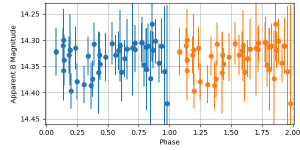(b)(c)(d)
 Figure 29. Phased light curves of (a) WISE J 202625.0+334224 in filter B, (b) ZTF J202615.90+334024.7 in filter B, (c) WISE J 202625.0+334224 in the r’ filter, (d) ZTF J202615.90+334024.7 in the r’ filter.

The data presented in Figure 29 shows the phased light curves of both WISE J 202625.0+334224 and ZTF J202615.90+334024.7 in the B and r’ filters, respectively. In (a), a sinusoidal curve typical of RRc’s can be observed, while in (c), the light curve resembles more closely a binary system with varying eclipse depths. Both (b) and (d) are curves presenting very little meaning.

Information regarding the periods, distances, temperatures, magnitudes, luminosities, and the radii of these target stars were calculated and obtained. For our first variable, WISE J202625.0+334224, we were able to determine a period of 0.54061 ± 0.00023 days, a distance of 1193.4 ± 38.7 parsecs, a temperature range of 4700K to 5000K, a luminosity range of approximately 2100 to 2700 times the luminosity of our Sun and a radius range of approximately 60 to 80 times that of our Sun. For the second variable, ZTF J202615.90+334024.7, a period of 3.592 ± 0.1146 days was calculated, a distance of 348.67 ± 2.83 parsecs, a temperature range of 4300K to 4700K, a luminosity range of 60 to 75 times that of our Sun and an estimated radius range of 12 to 15 times the radius of our Sun. These results, as had both stars’ phased light curves, were not distinctive enough to obtain classifications.

It should be noted that even though these findings and results do not present sufficient context to classify the variable systems, conducting more observations and devoting further time to the analysis of the results could lead us to more conclusive findings.

Conclusions could not be drawn as to whether either variable star was an RRc Lyrae or a binary system (be it RS CVn or a By Draconis). This could in part be due to limitations in our data collection, such as, weather, atmospheric interference, insufficient information about metallicity, and limited telescope time. WISE J202625.0+334224 may have been given different classifications in the WISE and ZTF catalogues due to the varying results from applying typical variable star classification techniques. Further research observations and analyses of the two target stars WISE J202625.0+334224 and ZTF J202615.90+334024.7 will be conducted.

WISE J184723.2+215838 and WISE J184737.2+215605 Eclipsing Binaries

By Gurpiar Gill and Zac Jalbert

In a narrow slice of the Hercules constellation, two eclipsing binaries sit. WISE J184723.2+215838, located at RA 281.846842 and Dec 21.97733542, and WISE J184737.2+215605,  located at RA 281.9052853 and Dec 21.93491929. These stars, along with their surrounding comparison stars, can be seen in Figure 31(a). The analysis revealed both of these stars to be EW-type eclipsing binaries.

WISE J184723.2+215838 has a phased period plot clearly indicating that it is a EW eclipsing binary, as seen in Figure 31(b). This star system is located roughly 1684 parsecs away from the earth. The period is 0.3578 days. The temperature sits in a range of 6100 K to 6600 K. The luminosity varies between 12 and 20 times the luminosity of our sun.

The phased period plot of WISE J184737.2+215605 was not as clearly classified as the other star, indicating the star could be either an RRc variable star or a EW eclipsing binary, so further analysis needed to be done. This plot can be seen in Figure 31(c). A magnitude of 1.6-2.0 indicated that this could not be a RRc variable, making this also a EW eclipsing binary. The period was found to be 0.6899 days at a distance of 782 parsecs. A temperature range of 6100 K to 6500 K was calculated, and a luminosity of 11 to 15 times our sun.

For WISE J184723.2+215838, the information on the AASVO VSX catalog is correct, so no revisions need to be made. However, for WISE J184737.2+215605, the star is categorized on the AASVO VSX catalog as a RRc or EC. This can now be clarified to be a EW, so a revision is to be submitted.

#### WISE J211229.8+371905 and WISE J211218.3+371721 by Daryl Janzen

Chen et al (2018) classified WISE J211229.8+371905 as an RRab Lyrae variable and WISE J211218.3+371721 as a W Ursae Majoris eclipsing binary (see Figure 28). The data collected in the present study showed that while both stars are clearly periodic variables, neither classification is actually correct.

The data presented in Figure 29 below indicate that both stars are likely Algol-type binary systems—detached binary stars that are mainly spherical in shape, which normally have clearly defined starts and ends to eclipses. In this regard, WISE J211229.8+371905 is a fairly run-of-the-mill Algol variable, with:

• similar amplitude eclipses, indicating the stars have similar luminosities and masses
• partial eclipses indicated by the peaked (as opposed to flat) minima
• fairly flat light curve, indicating the stars are roughly spherically shaped and there are no significant reflection effects due to proximity.

In contrast, WISE J211218.3+371721 (Figure 30) is a more interesting target. Looking closely, the start and end of the primary eclipse are visible at phase 0.89 and 1.11, respectively. However, outside the eclipse the light curve has an asymmetric variability which peaks at phase 0.27. This may be due to spots on one of the stars which remain in a constant location due to tidal locking of the two stars, or could be due to gas streaming between the stars. Note that the additional set of V magnitude values taken from the ASAS-SN survey indicate that the maximum leading into the primary eclipse may be variable for this star system, indicating variability in any of these potential causes. Furthermore, the secondary eclipse is very shallow, indicating the one star is far less luminous, and the fact that the secondary eclipse midpoint is at phase 0.58 indicates fairly elliptical orbits.

Photometric data, combined with Gaia data that give a distance of more than 3300 light years, indicate that the WISE J211229.8+371905 system has a luminosity ranging from 52 times to 62 times the luminosity of our Sun as the stars come in and out of eclipse, while the temperature of the system remains at roughly 6200 K throughout the period of variability. As these two stars are not much hotter than our Sun, the much greater luminosity of the system indicates that they are giant stars which have depleted their core hydrogen fuel supply and moved on to a later stage of evolution.

The  WISE J211218.3+371721 system has roughly the same temperature of 6200K, and is about 2/3 the distance, at just over 2300 light years. However, the system’s luminosity is considerably less, as indicated by the higher magnitudes in Figure 30, with values ranging from 2.4 to 3.6 solar luminosities. These two stars are therefore likely main-sequence stars, with hydrogen-burning cores like our Sun.

#### References

Ballesteros, F.J., 2012, EPL, 97 34008

Chen X., Wang S., Deng L. et al 2018 ApJ 237 28

Chen, X., Wang, S., Deng, L., et al. 2020, ApJS, 249, 18

Drake A.J., Graham M.J., Djorgovski S. G. et al 2014 ApJ 13 9

Jayasinghe T., et al 2018 MNRAS 477 3 3145-3163

Udovichenko, S. N., 2007, OAP, 20, 217